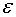11. Singular perturbation - when does regular perturbation not work?

Trouble might occur for instance when

1) the highest order derivative is multiplied by.

2) the problem totally changes characteristics when the parameteris equal to zero.

3) the problem is defined on infinite regions.

4) singular points are present.

5) the equation models physical processes with several time- or length scales.

1-5 are called singular perturbation problems.

In many cases we deal with problem containing boundary layers. We can roughly treat these problems by

i) lettingwe get a good approximation for the outer region.

ii) rescale the problem to get an inner approximation.

iii) match inner and outer approximations.

Singular perturbation is matched asymptotic expansion.